# Complex Analysis : Applications of Harmonic Functions

## Example Questions

### Example Question #52 : Complex Analysis

If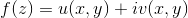and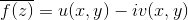are both analytic through a domain, which of the following is true?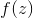must be constant throughoutis nowhere differentiable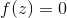is nowhere analyticmust be constant throughoutExplanation:

Sinceis analytic, the Cauchy-Riemann Equations give us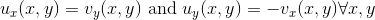Sinceis analytic, the Cauchy-Riemann Equations give us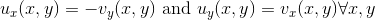.

Putting these two results together gives us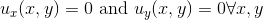which implies that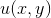is constant. Similar reasoning gives is that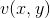is constant. This implies thatmust be constant throughout.

### All Complex Analysis Resources